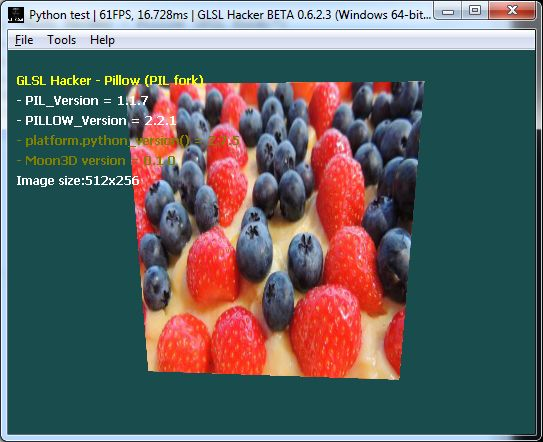# Beginning with Pillow, the PIL Fork (Python Imaging Library)

Article index:

## 4 – Reading the pixels

You can access to the individual pixels of the pixmap using the getpixel() function of the image object. In the following example, tex01 is a GLSL Hacker texture and pixels of Pillow image object are copied into tex01 using gh_texture.set_texel_2d() function.

The getpixel() function returns the value of a single pixel. Just give a tuple with the X and Y coordinates and getpixel() returns a 3-tuple RGB for a RGB image or a single value for a luminance image.

```if (im != None):
tex01 = moon3d.image.create2dRgbU8(imageW, imageH)

if (im.mode == "RGB"):
for y in range(0, imageH):
for x in range(0, imageW):
offset = y*imageW + x
xy = (x, y)
rgb = im.getpixel(xy)
gh_texture.set_texel_2d(tex01, x, y, rgb, rgb, rgb, 255)

elif (imout.mode == "L"):
for y in range(0, imageH):
for x in range(0, imageW):
offset = y*imageW + x
xy = (x, y)
r = im.getpixel(xy)
gh_texture.set_texel_2d(tex01, x, y, r, r, r, 255)
else: